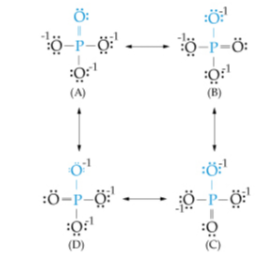# Problem: The bond order for a molecule is the number of bonds between a pair of atoms. For example, for the oxygen molecule, O = O, the bond order is two; for the hydrogen molecule, H - H, the bond order is one. Bond order indicates the strength of the bond. Consider the four resonating structures of the phosphate ion: Calculate the average bond order for a P - O bond (such as the one shown in blue) in a phosphate ion. Express your answer numerically as a decimal or improper fraction.

###### FREE Expert Solution
96% (436 ratings)
###### FREE Expert Solution
• For this problem, we are asked for the average bond order of P-O bond

• From the paragraph, it tells us that a single bond is equal to bond order of 1 while 2 bonds/double bonds is equal to bond order of 2

96% (436 ratings)###### Problem Details

The bond order for a molecule is the number of bonds between a pair of atoms. For example, for the oxygen molecule, O = O, the bond order is two; for the hydrogen molecule, H - H, the bond order is one. Bond order indicates the strength of the bond. Consider the four resonating structures of the phosphate ion: Calculate the average bond order for a P - O bond (such as the one shown in blue) in a phosphate ion. Express your answer numerically as a decimal or improper fraction.What scientific concept do you need to know in order to solve this problem?

Our tutors have indicated that to solve this problem you will need to apply the Resonance Structures concept. You can view video lessons to learn Resonance Structures. Or if you need more Resonance Structures practice, you can also practice Resonance Structures practice problems.

What is the difficulty of this problem?

Our tutors rated the difficulty ofThe bond order for a molecule is the number of bonds between...as medium difficulty.

How long does this problem take to solve?

Our expert Chemistry tutor, Sabrina took 1 minute and 53 seconds to solve this problem. You can follow their steps in the video explanation above.

What professor is this problem relevant for?

Based on our data, we think this problem is relevant for Professor Maxwell's class at UCF.